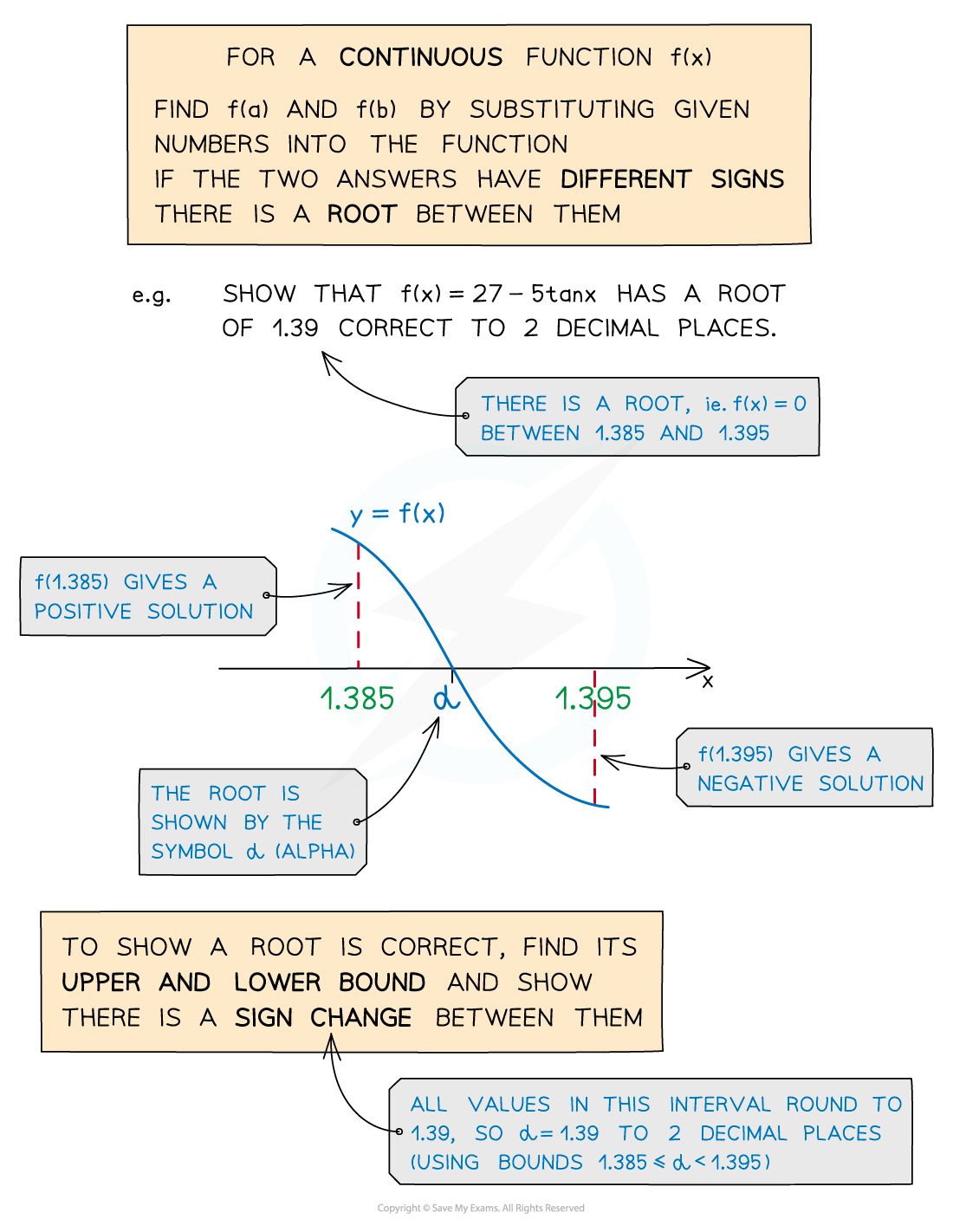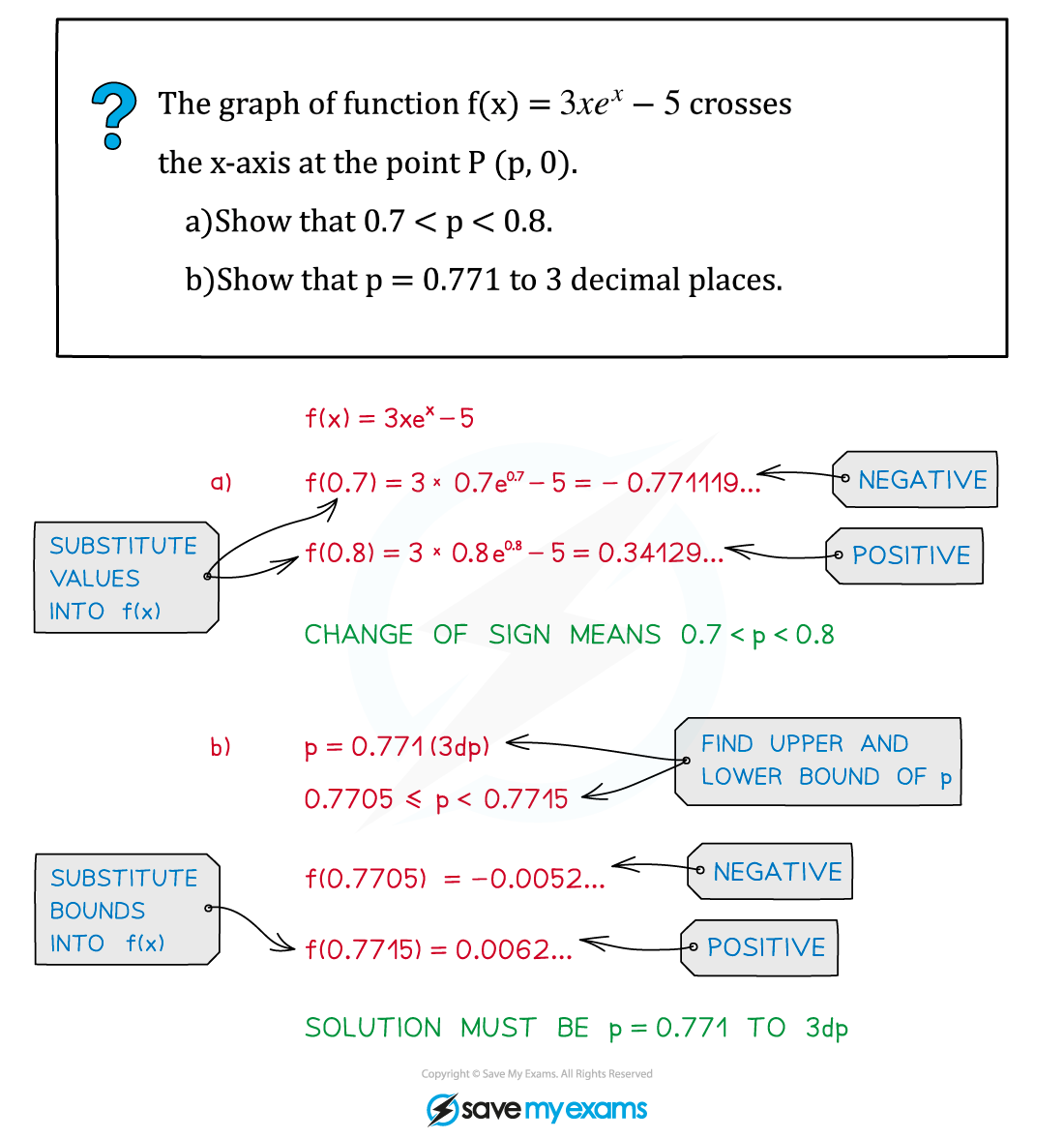# AQA A Level Maths: Pure复习笔记10.1.1 Change of Sign

### Change of Sign

#### What does a change of sign mean?

• sign change between f(a) to f(b) means there must be a root between a and b
• When an equation cannot be solved using the usual analytical methods, we can use change of sign to find approximate solutions
• We can say a root lies within a particular interval or is correct to a given accuracy
• Bounds can be used to show a root is correct
• Using sign change to find a root is only appropriate for continuous functions in a small interval#### Exam Tip

• Remember this will only work if the function is continuous and the interval is small enough.
• Sign change questions may be part of bigger numerical methods questions.

#### Worked Example